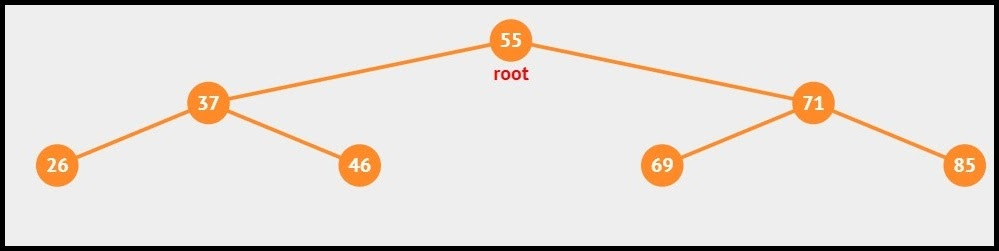# Construct a BST from given postorder traversal using Stack

## Introduction

In this article, we will learn how to construct a BST from a given postorder traversal using Stack. Such problems are really important to study and understand as they help us understand various concepts about Binary Trees

Once you go through this article carefully, you will better understand Binary Search TreesTree Traversals, and Stack

## Problem Statement

Your task is to create a Binary Search Tree from a given Post Order Traversal using Stack. Print the Inorder Traversal of the BST.

## Example

Consider the given Post Order Traversal: {26, 46, 37, 69, 85, 71, 55}
The Inorder Traversal that should be printed is: {26, 37, 46, 55, 69, 71, 85}
The BST formed looks like this:(source: BST Visualizer)

Recommended: Try the question yourself first before moving on to the approach and solution code.

## Approach and Explanation

To solve the problem at hand, we have to perform Inorder Traversal on the given Post Order array. The point of highlight is that, during Post Order traversal, the root node is always found at the end of the array. So keeping this in mind, we get the root of our BST. Now iteratively find the left and right child for each node.

The approach in this article discusses how to construct the BST from a given Post Order traversal array.

• Create a function to construct the BST (say constructBST). This function will take the input array, which is the post order traversal, from the user. Let’s name it postOrder. The function also takes the size of this array.

• Declare a variable to store the root node (say rootNode). Set the value for rootNode to the last element of postOrder (that is postOrder[size-1]).

• Create a stack (say bstStk) that will act as a buffer and hold the potential parent nodes.

• Push the rootNode into the stack.

• Now iterate through the postOrder array in reverse order. For each iteration, do:
• Create a node (say x) of the element at postOrder[i]

• Create a temporary node (say tempNode) and set its value to NULL.

• Now iterate while the stack is not empty and the element at postOrder[i] is less than the element at the top of the stack.
• If true, then set the value of tempNode as the top of the stack and pop that element from the stack.

• Once the while loop is completed, check if the value of tempNode is null or not.
• If null then, set x as the right child of the element at the top of the stack.
• If not null then, set x as the left child of tempNode.

• Finally, push x into the stack.

• Once the for loop is exhausted, print the root node. Call the printInorder() function and pass rootNode so as to print the inorder traversal of the newly constructed BST.

## Java Implementation

``````import java.util.Stack;

class BSTNode {
int data;
BSTNode leftChild, rightChild;

BSTNode(int data)
{
this.data = data;
leftChild = rightChild = null;
}
}

public class StackToBST {

void constructBST(int[] postOrder, int size)
{
BSTNode rootNode = new BSTNode(postOrder[size - 1]);
Stack<BSTNode> bstStk = new Stack<>();
bstStk.push(rootNode);

for (int i = size - 2; i >= 0; --i) {

BSTNode x = new BSTNode(postOrder[i]);

BSTNode tempNode = null;
while (!bstStk.isEmpty() && postOrder[i] < bstStk.peek().data) {
tempNode = bstStk.pop();
}

if (tempNode == null) {
bstStk.peek().rightChild = x;
} else {
tempNode.leftChild = x;
}
bstStk.push(x);
}

System.out.println("The Root node is: " + rootNode.data);

System.out.println("INORDER TRAVERSAL OF THE BST: ");
printInorder(rootNode);

}

void printInorder(BSTNode node)
{
if (node == null)
return;
printInorder(node.leftChild);
System.out.print(node.data + " ");
printInorder(node.rightChild);
}

public static void main(String[] args)
{
StackToBST tree = new StackToBST();
int[] postOrder = new int[] { 26, 46, 37, 69, 85, 71, 55};
int size = postOrder.length;

tree.constructBST(postOrder, size);
}
}``````

OUTPUT

``````The Root node is: 55
INORDER TRAVERSAL OF THE BST:
26 37 46 55 69 71 85 ``````

## Complexities

### Time Complexity

In the given approach, we select each element from the Post Order array and compare it with the top of the stack.

We use a loop of length ‘n’ to traverse the postorder array, and in each iteration, depending upon the value of the current element and those present in the stack, we perform push or pop operations. At first look, the complexity seems to be more than O(n).

But if you observe carefully, every element in the postorder array is pushed into and popped from the stack at most once. So, the total number of operations is roughly 2*n, and time complexity turns out to be O(2*n) which is O(n) only.

T(n) = O(n),

where n is the size of the BST.

### Space Complexity

In the given approach, we create a stack whose space is proportional to the height of the given BST at any instance.

Thus,

Space Complexity = O(h),

where h is the height of the BST.

1. What is a BST?
Binary Search Tree or BST is a special kind of Binary Tree where each node has two child nodes, and the nodes in the left subtree are smaller than the nodes in the right subtree. To know more about trees, visit our CN Library | Free Online Coding Resources

2. What is the difference between Post Order and Inorder Traversal?
The order of traversal in Post Order Traversal is left, right, root. The order of traversal in Inorder Traversal is left, root, right. To know more about tree traversals visit, Traversals

## Key Takeaways

To summarize the article, we discussed how to construct a BST from a given postorder traversal using Stack. We saw the problem statement and an example. Then we saw an approach to our problem followed by a Java implementation and its time and space complexities. In the end, we discussed a few FAQs.

Want to improve your coding skills? Start practicing problems of various difficulty levels on our CodeStudio.

Want to learn about topics like Web Technologies, Programing Fundamentals, Aptitude, DSA, and much more? Visit CN Library | Free Online Coding Resources today.

Happy Coding!